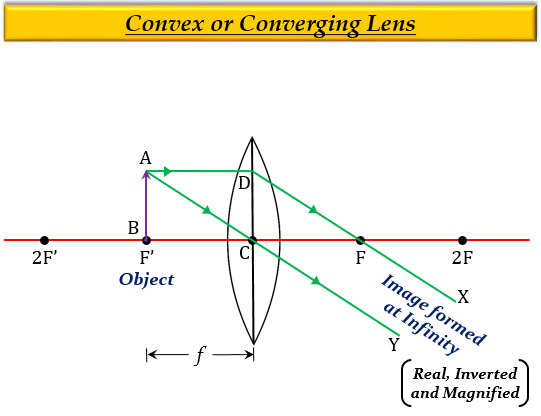# If an object is placed at the focus of a convex lens, where is the image formed?

If an object is placed at the focus of a convex lens, then the image is formed at infinity.

Explanation

When an object is at the focus $(F')$ of a convex lens, which means the object is at a distance equal to the focal length $f$ of a convex lens, the image formed is real, inverted, highly enlarged (magnified), and at infinity.Updated on: 10-Oct-2022

2K+ Views